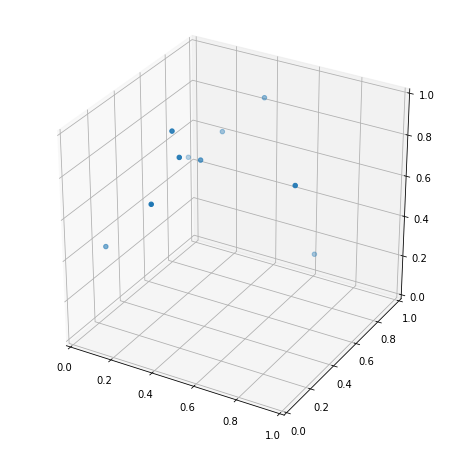# 3D axes set_aspect(“equal”) NotImplementedError

NotImplementedError: It is not currently possible to manually set the aspect on 3D axes

Bug summary
I am making a 3d scatter plot in jupyter notebook, when I try to change the axis aspect an error raises.

Code to reproduce the error

``````%%matplotlib notebook

import matplotlib.pyplot as plt
import numpy as np
from mpl_toolkits.mplot3d import Axes3D

x, y, z = list(np.random.rand(3,10))

fig = plt.figure( figsize=(8,8))
ax = fig.add_subplot(111, projection='3d')
ax.scatter(x,y,z)
ax.set_aspect("equal")
``````

Actual outcome

``````---------------------------------------------------------------------------
NotImplementedError                       Traceback (most recent call last)
<ipython-input-1-2c7b67cedd81> in <module>
11 ax = fig.add_subplot(111, projection='3d')
12 ax.scatter(x,y,z)
---> 13 ax.set_aspect("equal")

/hpcfs/apps/anaconda/3.7/lib/python3.7/site-packages/matplotlib/axes/_base.py in set_aspect(self, aspect, adjustable, anchor, share)
1279         if (not cbook._str_equal(aspect, 'auto')) and self.name == '3d':
1280             raise NotImplementedError(
-> 1281                 'It is not currently possible to manually set the aspect '
1282                 'on 3D axes')
1283

NotImplementedError: It is not currently possible to manually set the aspect on 3D axes```
``````

Expected outcome

A plot with axis ratio 1:1:1Matplotlib version

• Operating system: Linux CentOS
• Matplotlib version: 3.1.0
• Matplotlib backend: nbAgg
• Python version: 3.7.3 (build h33d41f4_1, channel conda-forge)
• Jupyter version: 7.8.0 (build py37h5ca1d4c_0, channel conda-forge)

I have installed python using conda, using the channel conda-forge.Full dicussion on github.com

## 2 thoughts on “3D axes set_aspect(“equal”) NotImplementedError”

1. I ran into this and had two options

1. `conda install matplotlib=2.2.3`
2. `# ax.set_aspect('equal')`
2. `ax.set_aspect('equal')` produces wrong output for 3D axes. It was therefore removed. If you want to get wrong output, you can revert to an old version of matplotlib and continue using it.